1-D Kinematics - Lesson 1 - Describing Motion with Words

# Speed and VelocityJust as distance and displacement have distinctly different meanings (despite their similarities), so do speed and velocity. Speed is a scalar quantity that refers to "how fast an object is moving." Speed can be thought of as the rate at which an object covers distance. A fast-moving object has a high speed and covers a relatively large distance in a short amount of time. Contrast this to a slow-moving object that has a low speed; it covers a relatively small amount of distance in the same amount of time. An object with no movement at all has a zero speed.

### Velocity as a Vector Quantity

Velocity is a vector quantity that refers to "the rate at which an object changes its position." Imagine a person moving rapidly - one step forward and one step back - always returning to the original starting position. While this might result in a frenzy of activity, it would result in a zero velocity. Because the person always returns to the original position, the motion would never result in a change in position. Since velocity is defined as the rate at which the position changes, this motion results in zero velocity. If a person in motion wishes to maximize their velocity, then that person must make every effort to maximize the amount that they are displaced from their original position. Every step must go into moving that person further from where he or she started. For certain, the person should never change directions and begin to return to the starting position.

Velocity is a vector quantity. As such, velocity is direction aware. When evaluating the velocity of an object, one must keep track of direction. It would not be enough to say that an object has a velocity of 55 mi/hr. One must include direction information in order to fully describe the velocity of the object. For instance, you must describe an object's velocity as being 55 mi/hr, east. This is one of the essential differences between speed and velocity. Speed is a scalar quantity and does not keep track of direction; velocity is a vector quantity and is direction aware.

###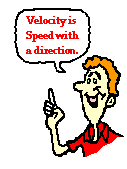Determining the Direction of the Velocity Vector

The task of describing the direction of the velocity vector is easy. The direction of the velocity vector is simply the same as the direction that an object is moving. It would not matter whether the object is speeding up or slowing down. If an object is moving rightwards, then its velocity is described as being rightwards. If an object is moving downwards, then its velocity is described as being downwards. So an airplane moving towards the west with a speed of 300 mi/hr has a velocity of 300 mi/hr, west. Note that speed has no direction (it is a scalar) and the velocity at any instant is simply the speed value with a direction.

### Calculating Average Speed and Average Velocity

As an object moves, it often undergoes changes in speed. For example, during an average trip to school, there are many changes in speed. Rather than the speed-o-meter maintaining a steady reading, the needle constantly moves up and down to reflect the stopping and starting and the accelerating and decelerating. One instant, the car may be moving at 50 mi/hr and another instant, it might be stopped (i.e., 0 mi/hr). Yet during the trip to school the person might average 32 mi/hr. The average speed during an entire motion can be thought of as the average of all speedometer readings. If the speedometer readings could be collected at 1-second intervals (or 0.1-second intervals or ... ) and then averaged together, the average speed could be determined. Now that would be a lot of work. And fortunately, there is a shortcut. Read on. The average speed during the course of a motion is often computed using the following formula: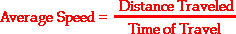In contrast, the average velocity is often computed using this formula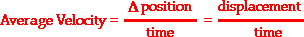Let's begin implementing our understanding of these formulas with the following problem:

Q: While on vacation, Lisa Carr traveled a total distance of 440 miles. Her trip took 8 hours. What was her average speed?

To compute her average speed, we simply divide the distance of travel by the time of travel.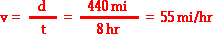That was easy! Lisa Carr averaged a speed of 55 miles per hour. She may not have been traveling at a constant speed of 55 mi/hr. She undoubtedly, was stopped at some instant in time (perhaps for a bathroom break or for lunch) and she probably was going 65 mi/hr at other instants in time. Yet, she averaged a speed of 55 miles per hour. The above formula represents a shortcut method of determining the average speed of an object.

### Average Speed versus Instantaneous Speed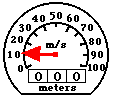Since a moving object often changes its speed during its motion, it is common to distinguish between the average speed and the instantaneous speed. The distinction is as follows.

• Instantaneous Speed - the speed at any given instant in time.
• Average Speed - the average of all instantaneous speeds; found simply by a distance/time ratio.

You might think of the instantaneous speed as the speed that the speedometer reads at any given instant in time and the average speed as the average of all the speedometer readings during the course of the trip. Since the task of averaging speedometer readings would be quite complicated (and maybe even dangerous), the average speed is more commonly calculated as the distance/time ratio.

Moving objects don't always travel with erratic and changing speeds. Occasionally, an object will move at a steady rate with a constant speed. That is, the object will cover the same distance every regular interval of time. For instance, a cross-country runner might be running with a constant speed of 6 m/s in a straight line for several minutes. If her speed is constant, then the distance traveled every second is the same. The runner would cover a distance of 6 meters every second. If we could measure her position (distance from an arbitrary starting point) each second, then we would note that the position would be changing by 6 meters each second. This would be in stark contrast to an object that is changing its speed. An object with a changing speed would be moving a different distance each second. The data tables below depict objects with constant and changing speed.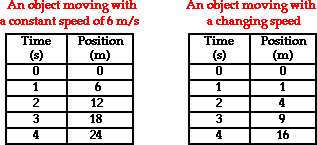Now let's consider the motion of that physics teacher again. The physics teacher walks 4 meters East, 2 meters South, 4 meters West, and finally 2 meters North. The entire motion lasted for 24 seconds. Determine the average speed and the average velocity.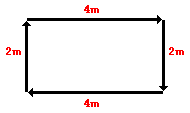The physics teacher walked a distance of 12 meters in 24 seconds; thus, her average speed was 0.50 m/s. However, since her displacement is 0 meters, her average velocity is 0 m/s. Remember that the displacement refers to the change in position and the velocity is based upon this position change. In this case of the teacher's motion, there is a position change of 0 meters and thus an average velocity of 0 m/s.

Here is another example similar to what was seen before in the discussion of distance and displacement. The diagram below shows the position of a cross-country skier at various times. At each of the indicated times, the skier turns around and reverses the direction of travel. In other words, the skier moves from A to B to C to D.Use the diagram to determine the average speed and the average velocity of the skier during these three minutes. When finished, click the button to view the answer.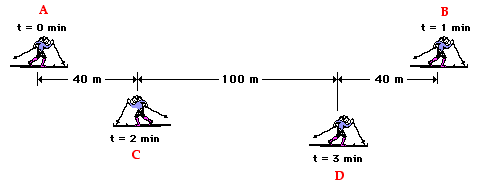As a last example, consider a football coach pacing back and forth along the sidelines. The diagram below shows several of coach's positions at various times. At each marked position, the coach makes a "U-turn" and moves in the opposite direction. In other words, the coach moves from position A to B to C to D.What is the coach's average speed and average velocity? When finished, click the button to view the answer.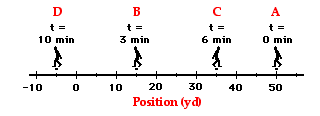In conclusion, speed and velocity are kinematic quantities that have distinctly different definitions. Speed, being a scalar quantity, is the rate at which an object covers distance. The average speed is the distance (a scalar quantity) per time ratio. Speed is ignorant of direction. On the other hand, velocity is a vector quantity; it is direction-aware. Velocity is the rate at which the position changes. The average velocity is the displacement or position change (a vector quantity) per time ratio.### We Would Like to Suggest ...Sometimes it isn't enough to just read about it. You have to interact with it! And that's exactly what you do when you use one of The Physics Classroom's Interactives. We would like to suggest that you combine the reading of this page with the use of our Name That Motion Interactive. It is found in the Physics Interactive section and allows a learner to apply concepts of speed, velocity and acceleration.

Visit Name That Motion.

Next Section: